McIDAS Programmer's Manual
Version 2015

# Scientific utilities

The McIDAS-X library provides a set of scientific utilities for computing meteorological parameters such as potential temperature, equivalent potential temperature, and mixing ratio.

The table below provides an alphabetical listing of the scientific utilities provided in McIDAS-X.

C function Fortran function Description

not available

lab

computes potential and equivalent potential temperature and mixing ratio, given the temperature, dew point, pressure, and station elevation

McBeta

mcbeta

computes beta parameter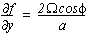McCape

mccape

computes Convective Available Potential Energy

McCoriolis

mccorfor

computes the coriolis parameter (f=2ΩsinΦ)

McDewpt

mcdewpt

computes dewpoint

McDirec

mcdirec

computes meteorological direction of wind for u- and v-components

McDiver

mcdiver

computes divergence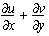McDivergeParm

mcdivergeparm

compute divergence of gridded parameter

McHeatIndex

mcheatindex

computes the heat index, given the temperature and dewpoint

McHelic

mchelic

computes helicity

McHypsoP

mchypsop

integrates hypsometric equation to compute a pressure at a given height

McHypsoZ

mchypsoz

integrates hypsometric equation to compute a new height at a given pressure

McLatentHeat

mclatvap

latent heat of vaporization

McLiftCLevel

mclcl

parcel temperature and pressure at LCL

McMixing

mcrmix

mixing ratio

McPFromTheta

mcpfromtheta

returns pressure when given theta and temperature

McRelativeHumidity

mcrelativehumidity

computes the relative humidity, given the temperature and dewpoint

McSatVapor

mcsatvap

saturation vapor pressure over water

McSatVaporIce

mcsatvapi

saturation vapor pressure over ice

McSheard

mcsheard

computes shear deformation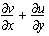McSndAnl

mcsndanl

computes various stability parameters, given a sounding

McSpeed

mcspeed

computes wind speed from u- and v-components

McStationPres

mcstnpres

station pressure, given altimeter and station elevation

McStretd

mcstretd

computes the stretching deformation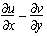McTDFromMixing

mctamr

computes dew point from mixing ratio

McTempAtThetae

mctasa

McTFromTheta

mctfromtheta

returns temperature when given theta and pressure

McTheta

mctheta

potential temperature

McThetae

mcthetae

equivalent potential temperature

McThetaw

mcthetaw

wet bulb potential temperature

McUandV

mcuandv

computes wind u- and v-components

McVirtTemp

mcvirttemp

virtual temperature

McVort

mcvort

computes vorticity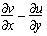McWetBulb

mcwetbulb

wet bulb temperature

McWindChill

mcwindchill

computes the wind chill, given the temperature and wind speed (old formula)

McWindChill2001

mcwindchill2001

computes the wind chill, given the temperature and wind speed (uses the 2001 algorithm)

not available

rmix

determines the mixing ratio, given the temperature and pressure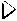For more information about these scientific functions, see the online man pages provided with the McIDAS-X software.

#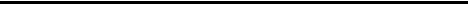Given the temperature, dew point and pressure, the lab function computes the following:

• Potential temperature
• Equivalent potential temperature
• Mixing ratio

To compute these meteorological parameters using the pressure at the station instead of the reported pressure, which has been corrected to sea level, the lab function will automatically adjust for the pressure differences due to elevation.

The following example computes potential and equivalent potential temperatures, and mixing ratio with adjustments made to the pressure based on the height.

 ``` double precision derived(3) ! derived parameters double precision dewpt ! dewpt in K double precision elev ! elevation in meters double precision miss ! missing data code double precision press ! pressure in mb double precision temp ! temperature in K temp = 292.d0 dewpt= 289.d0 press=1018.29 miss=1.e35 elev=214 C the adjustment based on elevation is done call lab(temp,dewpt,press,elev,1,miss,derived,3) C this will return theta-e in derived(1), theta in derived(1) C and mixing ratio in derived(3) C the values returned will be: C derived(1) = 323.115 C derived(2) = 292.612 C derived(3) = 11.521 C the adjustment based on elevation is not done call lab(temp,dewpt,press,elev,0,miss,derived,3) C the values returned will be: C derived(1) = 319.961 C derived(2) = 290.491 C derived(3) = 11.226 ```

#Given the temperature and dew point, the functions McHeatIndex and mcheatindex will compute the heat index. The value returned will be given in the same units as the input temperature. Below is an example of the function mcheatindex.

 ``` integer ok double precision temperature double precision dewpoint double precision heatindex temperature = 30.d0 dewpoint = 20.d0 ok = mcheatindex (temperature, dewpoint, 'C', heatindex) if (ok .lt. 0)then call sdest('error calculating the heat index',0) return endif ``` ```c--- upon successful completion the value of heatindex will c--- be 32.5 degrees Celsius ```

#Given the temperature and dew point, the functions McRelativeHumidity and mcrelativehumidity will compute the relative humidity. The value returned will be given in the dimensionless units of percentage. Below is an example of the function mcrelativehumidity.

 ```integer ok double precision temperature double precision dewpoint double precision rh ``` ``` temperature = 30.d0 dewpoint = 20.d0 ``` ``` ok = mcrelativehumidity (temperature, dewpoint, 'C', rh) if (ok .lt. 0)then call sdest('error calculating the relative humidity',0) return endif ``` ```c--- upon successful completion the value of rh will be 55.1 ```

#Given the temperature and wind speed, the functions McWindChill and mcwindchill will compute the wind chill. The value returned will be given in the same units as the input temperature. Below is an example of the mcwindchill function.

 ``` integer ok double precision temperature double precision windspeed double precision windchill ``` ``` temperature = 20.d0 windspeed = 10.d0 ``` ``` ok = mcwindchill (temperature, windspeed, 'F', 'MPS', & windchill) ``` ``` if (ok .lt. 0)then call sdest('error calculating the wind chill',0) return endif ``` ```c---upon successful completion the value of windchill will c---be -15 degrees Fahrenheit ```

#Given a temperature, in Kelvin, and the pressure, in mb, the rmix function will return the mixing ratio. The returned value, ws, is defined as the mass of water vapor per mass of dry air. It is dimensionless, with units of g/kg. The sample code below computes the saturation mixing ratio at 0° Celsius for all pressures 100, 150, 200, 250, ... 950, 1000 mb, resulting in 40.44, 26.39, 19.59, 15.57, ... 4.02, 3.82 g/kg.

 ``` integer i real temperature real pressure real mix temperature=273.15 do 100 i=1000,100,-50 pressure=float(i) mix=rmix(temperature,pressure) 100 continue ```

#Given vertical profiles of pressure, temperature, dew point, wind speed and direction, the sndanl function computes these stability indices:

• Parcel dew point, potential temperature, equivalent potential temperature and mixing ratio
• Precipitable water
• Convective temperature and forecast maximum temperature (for data from 1200 UTC only)
• Lifted index, total-totals index, K-index and sweat index
• Equilibrium pressure level

Below is an example of the sndanl function.

 ``` parameter (NLEV = 9) real press(NLEV) real temp(NLEV) real dewpt(NLEV) real dir(NLEV) real spd(NLEV) real stabil(12) c--- get sounding data : c--- assume the arrays; press, temp, dewpt, dir and spd c--- have been initialized with the following values c--- press(mb) temp(K) dewpt(K) dir(DEG) spd(MPS) c--- 988.0 300.0 299.8 250 1.5 c--- 925.0 303.0 280.0 270 3.8 c--- 850.0 297.7 269.0 285 5.6 c--- 700.0 277.5 276.1 290 12.3 c--- 500.0 262.1 252.1 265 13.3 c--- 400.0 253.6 240.5 280 29.8 c--- 300.0 237.6 226.0 280 33.4 c--- 250.0 229.7 215.7 285 41.6 c--- 200.0 216.3 206.3 285 45.7 c--- perform the sounding analysis for 00Z call sndanl (0, NLEV, press, temp, dewpt, dir, spd, stabil) c--- upon return, the values stored in stabil will be as follows: c--- stabil(1) 282.4 parcel dewpoint (k) c--- stabil(2) 301.1 potential temperature (k) c--- stabil(3) 324.1 equivalent potential temperature (k) c--- stabil(4) 8.3 mixing ratio (g/kg) c--- stabil(5) 23.7 precipitable water (mm) c--- stabil(6) 32.7 convective temperature (c) c--- stabil(7) 26.9 forecast maximum temperature (c) c--- stabil(8) 2.7 lifted index (k) c--- stabil(9) 33.4 total totals c--- stabil(10) 445.5 equivalent pressure (mb) c--- stabil(11) 20.6 k index c--- stabil(12) 47.6 sweat index ```